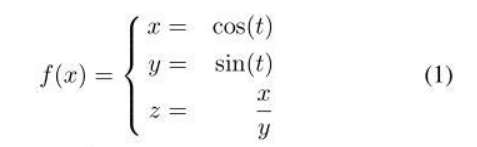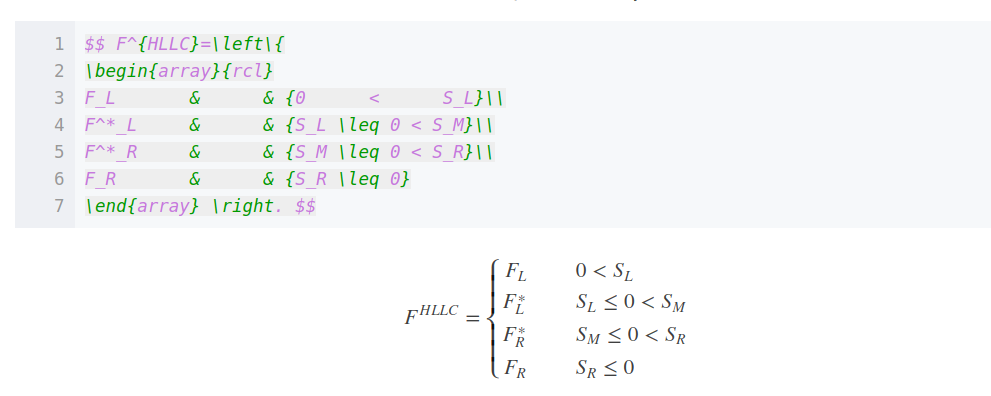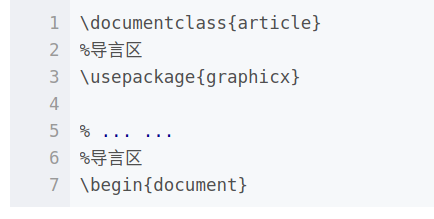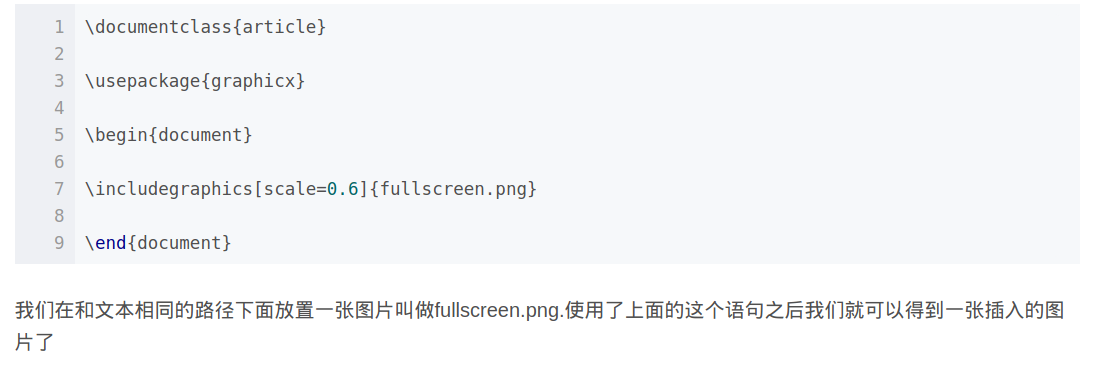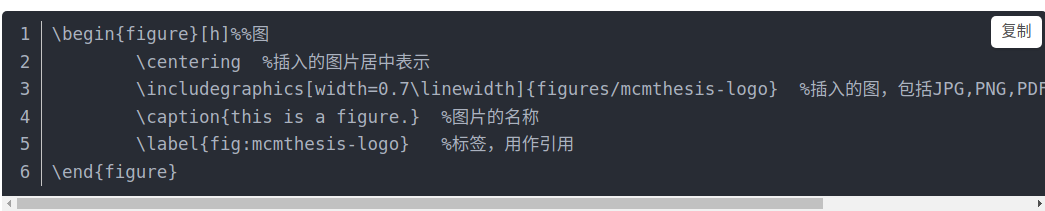# 下方輸入：

### \min\limits_{1<x<2}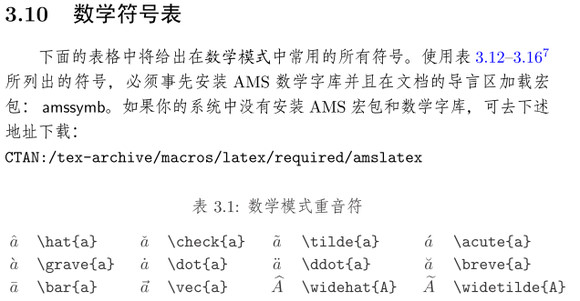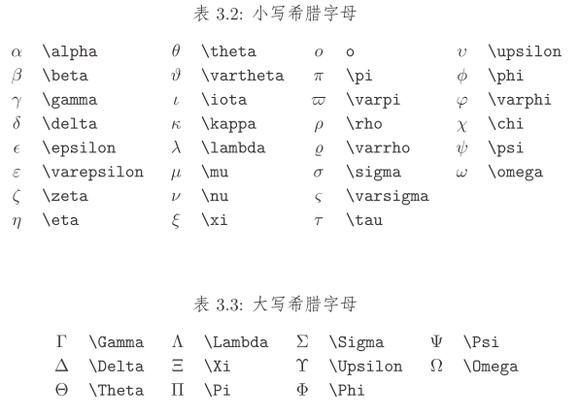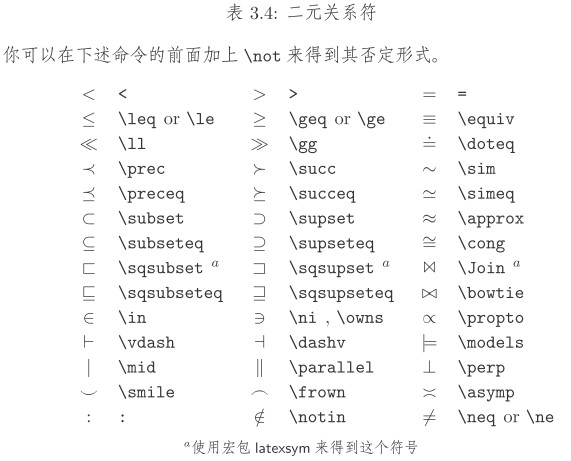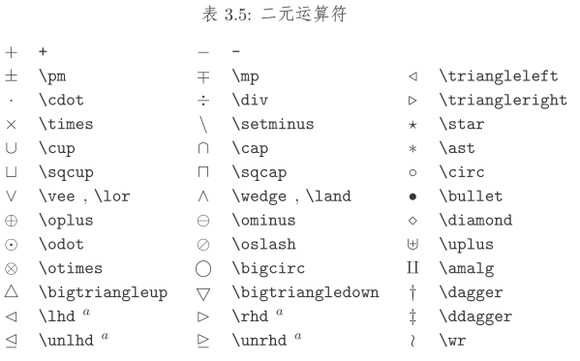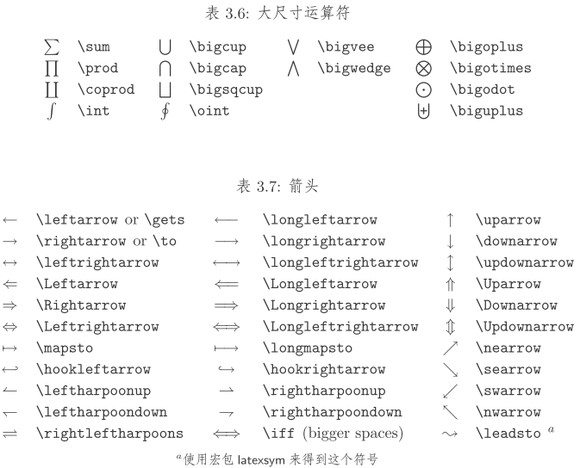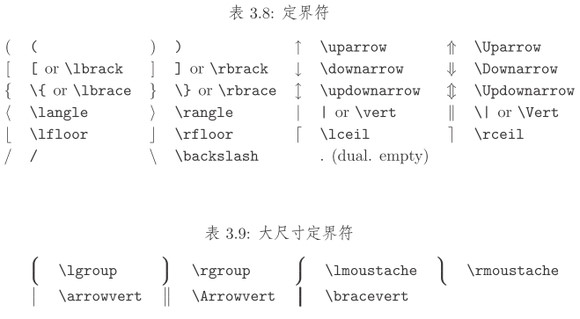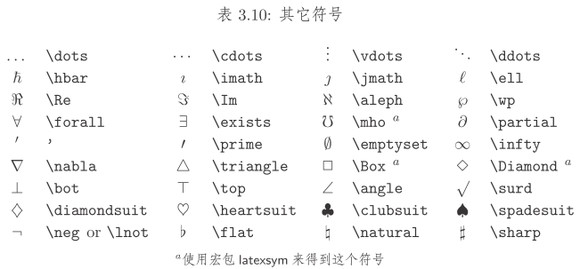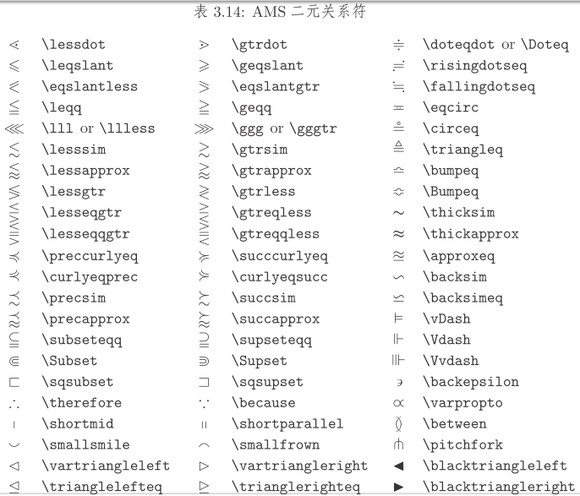\sqrt{2}

# 分段函式的大括號：

\begin{equation}

f(x)=\left\{

\begin{aligned}

x & = & \cos(t) \\

y & = & \sin(t) \\

z & = & \frac xy

\end{aligned}

\right.

\end{equation}